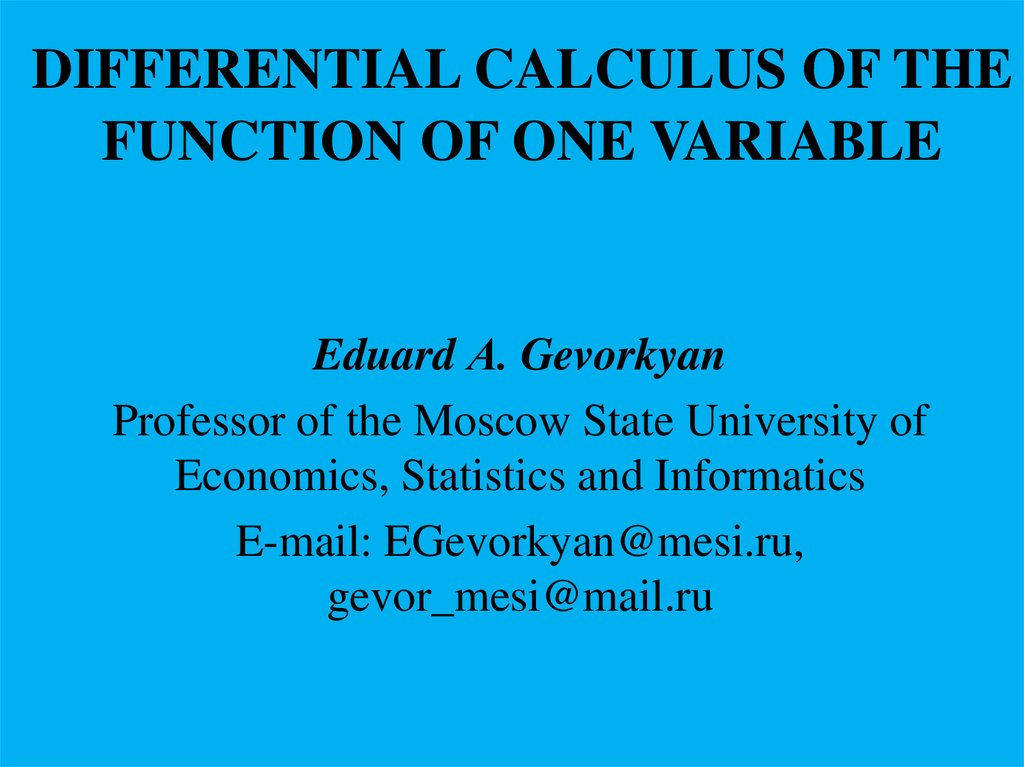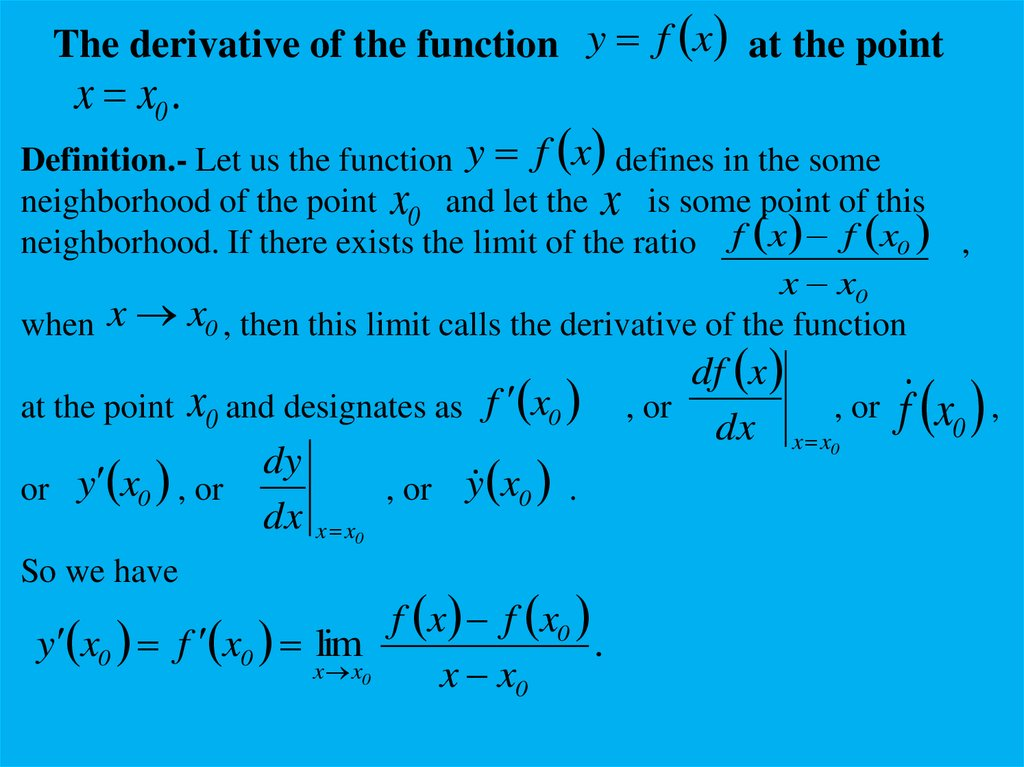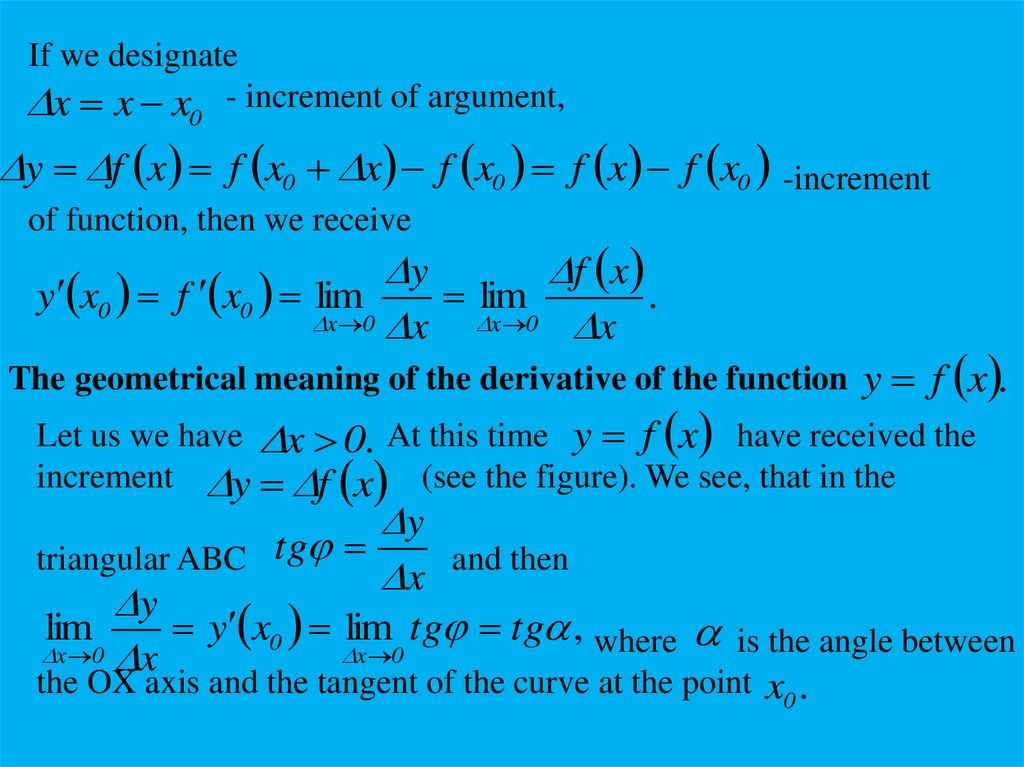# Differential calculus of the function of one variable

## 1. DIFFERENTIAL CALCULUS OF THE FUNCTION OF ONE VARIABLE

Eduard А. Gevorkyan
Professor of the Moscow State University of
Economics, Statistics and Informatics
E-mail: [email protected],
[email protected]

## 2.

The derivative of the function y f x at the point
x x0 .
Definition.- Let us the function y f x defines in the some
neighborhood of the point x0 and let the x is some point of this
neighborhood. If there exists the limit of the ratio f x f x0 ,
x x0
when x x0 , then this limit calls the derivative of the function
at the point x0 and designates as f x0
or y x0 , or
So we have
dy
dx
, or y x0 .
x x0
f x f x0
y x0 f x0 lim
.
x x0
x x0
df x
, or
, or
dx x x0
f x0 ,

## 3.

If we designate
x x x - increment of argument,
0
y f x f x0 x f x0 f x f x0 -increment
y
f x
y x0 f x0 lim
lim
.
x 0 x
x 0 x
The geometrical meaning of the derivative of the function y f x .
Let us we have x 0. At this time y f x have received the
increment y f x (see the figure). We see, that in the
y
triangular ABC tg
x and then
y
lim
y x0 lim tg tg , where is the angle between
x 0 x
x 0
the OX axis and the tangent of the curve at the point x0 .

## 4.

So we can say: the geometrical meaning of the derivative of the
y f x at the point x0 is the tangent of the angle
between the OX axis and the tangent of the curve at the point x0 .
The physical meaning of the derivative of the function S S t .
It is the instantaneous velocity at the moment t t0 when we have
the nonuniform motion (v const , v S ) , that is
t
dS t
vt St t0
, where S t is the distance covered.
dt t t0
t
The equation of the tangent of the curve at the point x0 .
Let y ktg x b
is the equation of the tangent of the curve at the
point x0 . As the point x0 ; f x0 belongs to the curve and the tangent
then we have the following system of equations
y ktg x b,
f x0 ktg x0 b.

## 5.

From this system taking into account that ktg f x0 we can receive
the equation for the tangent in the form y f x f x x x .
0
0
Unilateral derivatives.
Let us y f x defines in the right-side neighborhood of the
point x0 and exists the limit
0
f x0 x f x0
lim
f x0 0 -right-side derivative
x 0 0
x
of the function y f x at the point x0 .
Similarly we can define the left-side limit of the function y f x
at the point x0 .
lim
x 0 0
f x0 x f x0
f x0 0 .
x

## 6.

Note, that if the following condition takes place at the point x0
f x0 0 f x0 0 then we say, that the function y f x
has the derivative at the point x0 , that is
f x0 0 f x0 0 f x0 .
Example 1.- f x x 2 . In this case, it is easy to understand that
f 0 0 f 0 0 f 0 0.
has the derivative at the point x 0.
x, x 0,
Here we have
f x x
x, x 0.
f 0 0 1, f 0 0 1. That is, this function at the point
Example 2.-
x 0 has no derivative.

## 7.

The difference form of the continuity condition.
Let us consider the continuity condition of the function y f x
at the point x x0 , that is lim f x f x0 , or
x x0
lim f x f x0 0, or
x x
x x0
0
x x0 x, x x0 x
lim f x f x0 0.
and we have
lim f x f x0 lim f x0 x f x0
x 0
x x0
lim f x lim y 0.
x 0
x 0
Differentiability of y f x .
Let y f x
defines in the interval a, b and
x a, b ; x a, b .

## 8.

Definition.- y f x at the point x is named differentiable if the
increment of this function y f x can be presented in a form
y f x A x x, where A A x , is some
constant, x and 0 when x 0.
Theorem.- for differentiability of y f x it is necessary and
sufficient, that it had finite derivative.
The proof of necessity.- Let the function y f x is differentiable,
that is y f x A x x, or
y
y
A x , lim
lim A lim x .
x 0 x
x 0
x 0
x
A const , lim x 0.
lim A A,
So we have
x 0
y
dy
lim
y x
f x A.
x 0 x
dx
x 0

## 9.

The proof of sufficiency.- Let it is given, that the function has finite
derivative, that is exists the limit
y
lim
y x f x .
x 0 x
y
It means, that the difference lim
f x x , x 0
x 0 x
when x 0. Then we have
Now if we denote f x A,
y f x x x x.
then we have
y A x x x, that required to be proved.
The differential of the function y f x .
Let us y f x is differentiable. At that time we have, that
y f x x x x.
The term f x x
function relative x.
is the linear part of the increment of the

## 10.

Note, that the quantities f x x and x are the infinitely small
of the same order. But the quantity x x in compared with
x is infinitely small of higher order, that is x x o x .
f x x is the leading linear part of the increase
So the term
of the y f x . The term f x x is named the differential
of the function y f x and is indicated as dy f x x.
Note, that if we have the function y x
dy dx x x x, that is dx x, So we have
dy df x
dy y dx f x dx y f x
.
dx
dx
So we can say, that the derivative of the function is equal to the
ratio of dy and dx. .

## 11.

Geometric meaning of the differential of the y f x .
Let us turn to the figure which was for the determine the derivative
of the function
dy
,
y f x . We know, that f x tg
dx
where is the angle between the curve and the tangent at the point x.
Then we have dy f x dx tg dx. On the other hand
KN KN
tg
tg , KN f x dx, KN dy.
MN
dx
So we can say, that the differential dy is the increase of
the ordinate of the tangent to the curve at the point x.

## 12.

The rules of calculation of derivatives of elementary functions.
Note, that all rules of calculating of derivatives of elementary
functions we can receive with help of definition of derivative of
the function.
1. The power function - y x n .
According to the definition of derivative we have
x lim
n
x 0
x x n x n .
x
Opening brackets using the binomial theorem, after some transformations
we get the following rule
n
n 1
x nx
.
x x
x
a
a
1. The indicative function - y a . a x lim
.
x 0
x
After some transformations we get
x
x
a a ln a.
x

## 13.

If a e, e x e x ln e e x .
3. Logarithmic function.-
1
1
y log a x, (log a x)
, a e, (ln x) .
x ln a
x
sin x
cos x, cos x sin x, (arcsin x)
1
,
1 x2
1
1
1
(arccos x)
, arctgx
, arcctgx
.
2
2
2
1 x
1 x
1 x